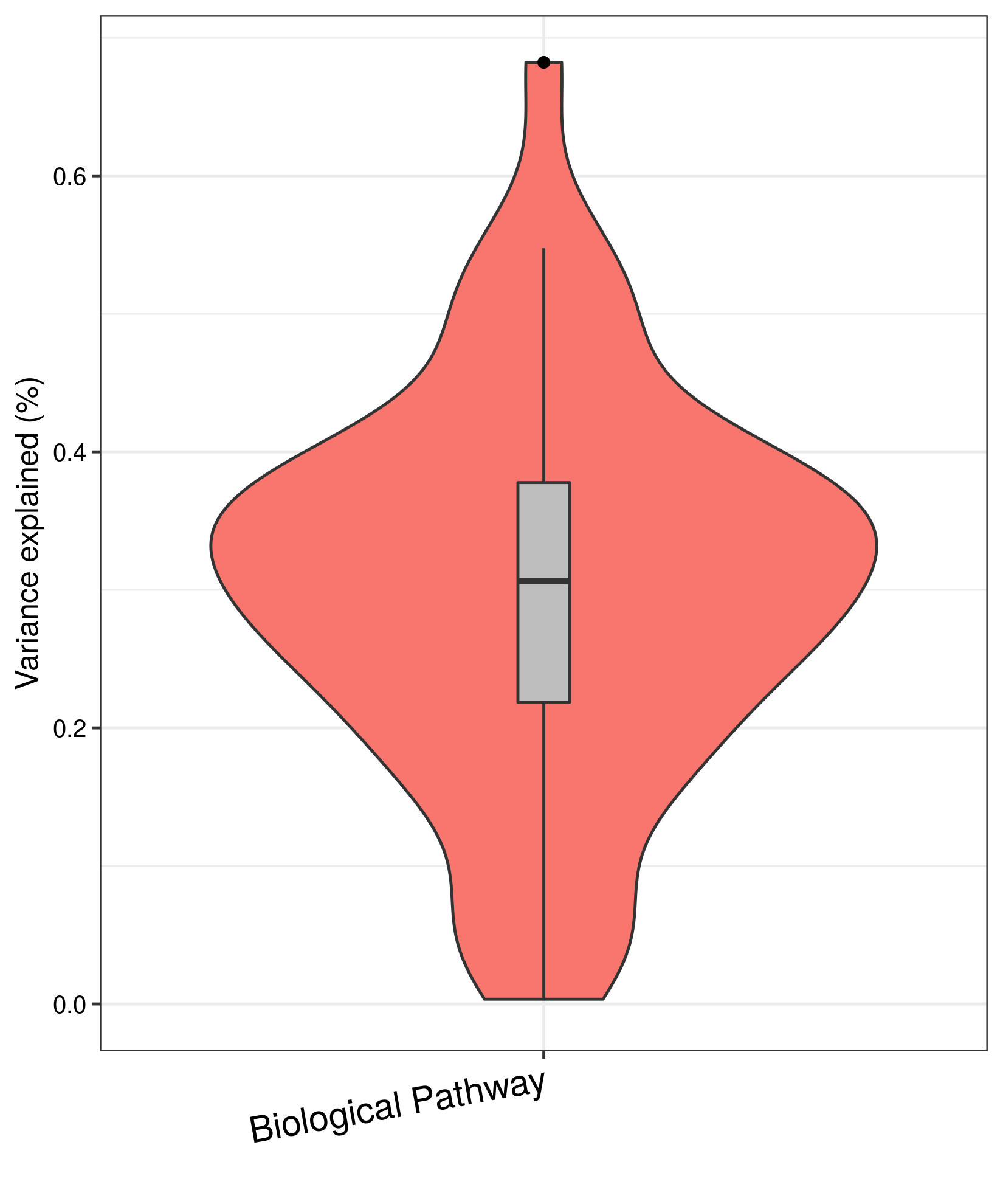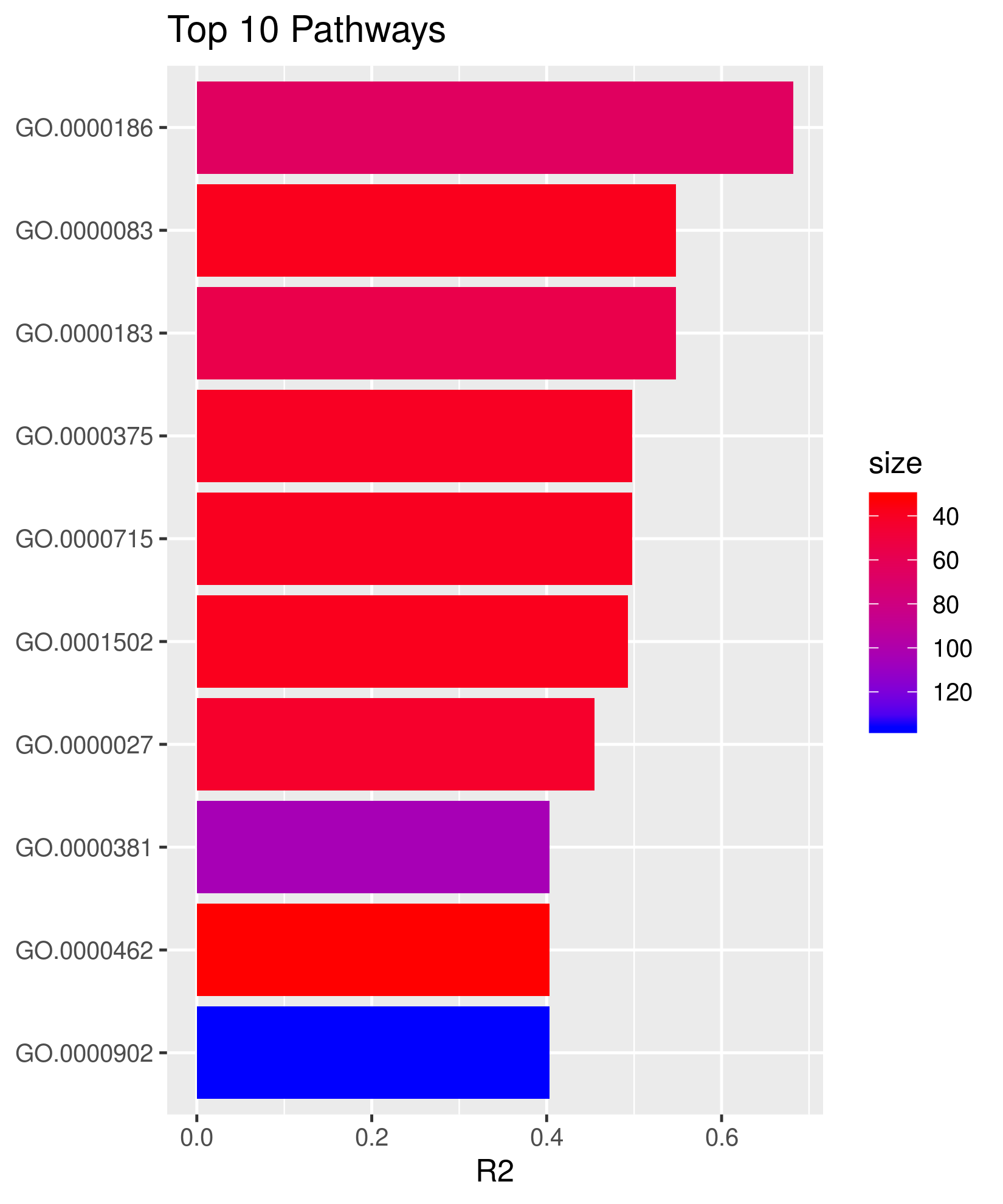# 1 Overview

## 1.1 Motivation

The identification of reproducible biological patterns from high-dimensional omics data is a key factor in understanding the biology of complex disease or traits. Incorporating prior biological knowledge into machine learning is an important step in advancing such research.

## 1.2 Deliverables

We have implemented a biologically informed multi-stage machine learing framework termed BioMM  specifically for phenotype prediction using omics-scale data based on biological prior information, for example, gene ontological pathways.

Features of BioMM in a nutshell:

1. Applicability for various omics data modalities.
2. Prioritizing outcome-associated functional patterns.
3. End-to-end prediction at the individual level based on biological pathway stratification.
4. Possibility for extension to machine learning models of interest.
5. Parallel computing.

# 2 Getting started

## 2.1 Installation

• Development version from Github (R 3.5):
``````install.packages("devtools")
library("devtools")
install_github("transbioZI/BioMM")``````
• Install BioMM from Bioconductor (R 3.6):
``````if (!requireNamespace("BiocManager", quietly = TRUE))
install.packages("BiocManager")
# The following initializes usage of Bioc devel
BiocManager::install(version='devel')
BiocManager::install("BioMM")``````

Note that, two different parallel computing strategies are implemented: For BioMM package available on Github, the parallel R package is implemented. The BiocParallel R package is employed in Bioconductor BioMM package which allows the use of parallel computation across any system.

``````library(BioMM)
library(BiocParallel)
library(parallel)
library(ranger)
library(rms)
library(glmnet)
library(e1071)
library(pROC)
library(vioplot)
library(variancePartition)
library(CMplot)``````

# 3 Omics data

A wide range of genome-wide omics data is supported for the use of BioMM including whole-genome DNA methylation, gene expression and genome-wide SNP data. Other types of omics data that can map into pathways are also encouraging.
For better understanding of the framework, we used a preprocessed genome-wide DNA methylation data with 26486 CpGs and 40 samples consisting of 20 controls and 20 patients. (0: healthy control and 1: patient) for demonstration.

``````## Get DNA methylation data
package="BioMM"))
``````##           label cg00000292 cg00002426 cg00003994 cg00005847
## GSM951223     1     0.0274     0.0029    -0.0027    -0.0196
## GSM951231     1     0.0644     0.0181    -0.0088     0.0057
## GSM951249     1    -0.0304    -0.0013    -0.0083    -0.0116
## GSM951273     1     0.0252     0.0039    -0.0091     0.0030
## GSM951214     1     0.0289    -0.0011    -0.0129     0.0173
## GSM951270     1     0.0635     0.0329     0.0184    -0.0023``````
``dim(studyData)``
``##     40 26487``

# 4 Feature stratification

Features like CpGs, genes or SNPs can be mapped into pathways based on genomic location and pathway annotation, as implemented in the function `omics2pathlist()`. The examples of pathway databases are gene ontology (GO), Reactome and KEGG, which are widely used public pathway repositories. Gene ontological pathway is used in this tutorial.

``````## Load annotation data
``````##           ID chr entrezID symbol
## 1 cg00000292  16      487 ATP2A1
## 2 cg00002426   3     7871  SLMAP
## 3 cg00003994   7     4223  MEOX2
## 4 cg00005847   2     3232  HOXD3
## 5 cg00006414   7    57541 ZNF398
## 6 cg00007981  11    24145  PANX1``````
``str(pathlistDB[1:3])``
``````## List of 3
##  \$ GO:0000002: Named chr [1:12] "291" "1890" "4205" "4358" ...
##   ..- attr(*, "names")= chr [1:12] "TAS" "IMP" "ISS" "IMP" ...
##  \$ GO:0000012: Named chr [1:11] "3981" "7141" "7515" "23411" ...
##   ..- attr(*, "names")= chr [1:11] "IDA" "IDA" "IEA" "IMP" ...
##  \$ GO:0000027: Named chr [1:31] "4839" "6122" "6123" "6125" ...
##   ..- attr(*, "names")= chr [1:31] "IMP" "IBA" "IBA" "IBA" ...``````
``````## Map to pathways (only 100 pathways in order to reduce the runtime)
pathlistDBsub <- pathlistDB[1:100]
pathlist <- omics2pathlist(data=studyData, pathlistDBsub, featureAnno,
restrictUp=100, restrictDown=20, minPathSize=10) ``````
``````##    Min. 1st Qu.  Median    Mean 3rd Qu.    Max.
##   12.00   38.00   50.50   60.84   74.25  164.00``````

# 5 BioMM framework

## 5.1 Recapitulation

Briefly, the BioMM framework consists of two learning stages . During the first stage, biological meta-information is used to ‘compress’ the variables of the original dataset into pathway-level ‘latent variables’ (henceforth called stage-2 data) using either supervised or unsupervised learning models. In the second stage, a supervised model is built using the stage-2 data with non-negative outcome-associated features for prediction.

## 5.2 End-to-end prediction modules

### 5.2.1 Interface to machine learning models

The end-to-end prediction is performed using `BioMM()` function. Both supervised and unsupervised learning are implemented in the BioMM framework, which are indicated by the argument `supervisedStage1=TRUE` or `supervisedStage1=FALSE`. Commonly used supervised classifiers: generalized regression models with lasso, ridge or elastic net regularization (GLM) , support vector machine (SVM)  and random forest  are included. For the unsupervised method, regular or sparse constrained principal component analysis (PCA)  is used. Generic resampling methods include cross-validation (CV) and bootstrapping (BS) procedures as the argument `resample1="CV"` or `resample1="BS"`. Stage-2 data is reconstructed using either resampling methods during machine learning prediction or independent test set prediction if the argument `testData` is provided.

#### 5.2.1.1 Example

To apply random forest model, we use the argument `classifier=randForest` in `BioMM()` with the classification mode at both stages. `predMode` indicate the prediction type, here we use classification for binary outcome prediction. A set of model hyper-parameters are supplied by the argument `paramlist` at both stages. Pathway-based stratification is carried out in this example. We focused on the autosomal region to limit the potential influence of sex on machine learning due to the phenomenon of X chromosome inactivation or the existence of an additional X chromosome in female samples. Therefore it’s suggested to exclude sex chromosome in the user-supplied `featureAnno` input file.

``````## Parameters
supervisedStage1=TRUE
classifier <- "randForest"
predMode <- "classification"
param1 <- MulticoreParam(workers = 1)
param2 <- MulticoreParam(workers = 10)
## If BioMM is installed from Github, please use the following assignment:
## param1 <- 1
## param2 <- 10

studyDataSub <- studyData[,1:2000] ## to reduce the runtime
set.seed(123)
result <- BioMM(trainData=studyDataSub, testData=NULL, pathlistDB, featureAnno,
restrictUp=100, restrictDown=10, minPathSize=10,
supervisedStage1, typePCA="regular",
resample1="BS", resample2="CV", dataMode="allTrain",
repeatA1=50, repeatA2=1, repeatB1=20, repeatB2=1,
nfolds=10, FSmethod1=NULL, FSmethod2=NULL,
cutP1=0.1, cutP2=0.1, fdr2=NULL, FScore=param1,
classifier, predMode,
paramlist, innerCore=param2)``````
``````##    Min. 1st Qu.  Median    Mean 3rd Qu.    Max.
##   10.00   10.00   11.00   11.98   13.00   22.00
##
##  Levels of predicted Y = 2
##
##      AUC   ACC    R2
## R2 0.875 0.875 0.627``````
``print(result)``
``````##      AUC   ACC    R2
## R2 0.875 0.875 0.627``````

Other machine learning models can be employed with the following respective parameter settings. For the classifier `"SVM"`, parameters can be tuned using an internal cross validation if `tuneP=TRUE`. For generalized regression model `glmnet`, elastic net is specified by the input argument `alpha=0.5`. Alternatively, `alpha=1` is for the lasso and `alpha=0` is the ridge. For the unsupervised learning `supervisedStage1=FALSE`, regular PCA `typePCA="regular"` is applied and followed with random forest classification `classifier2=TRUE`.

``````## SVM
supervisedStage1=TRUE
classifier <- "SVM"
predMode <- "classification"
gamma=10^(-3:-1), cost=10^(-3:1))

## GLM with elastic-net
supervisedStage1=TRUE
classifier <- "glmnet"
predMode <- "classification"
paramlist <- list(family="binomial", alpha=0.5,
ypeMeasure="mse", typePred="class")

## PCA + random forest
supervisedStage1=FALSE
classifier <- "randForest"
predMode <- "classification"

### 5.2.2 Interface to biological stratification strategies

For stratification of predictors using biological information, various strategies can be applied. Currently, `BioMM()` integrates gene ontological pathway based stratification, which not only accounts for epistasis between first level variables within the functional category, but also considers the interaction between pathways. Therefore, this may provide better information on functional insight.

#### 5.2.2.1 Example

End-to-end prediction based on pathway-wide stratification on genome-wide DNA methylation data is demonstrated below. PCA is used at stage-1 to reconstruct pathway level data, then the random forest model with 10-fold cross validation is applied on stage-2 data to estimate the prediction performance.

``````## Parameters
supervisedStage1=FALSE
classifier <- "randForest"
predMode <- "classification"
param1 <- MulticoreParam(workers = 1)
param2 <- MulticoreParam(workers = 10)
## If BioMM is installed from Github, please use the following assignment:
## param1 <- 1
## param2 <- 10

set.seed(123)
result <- BioMM(trainData=studyData, testData=NULL,
pathlistDBsub, featureAnno,
restrictUp=100, restrictDown=10, minPathSize=10,
supervisedStage1, typePCA="regular",
resample1="BS", resample2="CV", dataMode="allTrain",
repeatA1=40, repeatA2=1, repeatB1=40, repeatB2=1,
nfolds=10, FSmethod1=NULL, FSmethod2=NULL,
cutP1=0.1, cutP2=0.1, fdr2=NULL, FScore=param1,
classifier, predMode, paramlist, innerCore=param2)``````
``````##    Min. 1st Qu.  Median    Mean 3rd Qu.    Max.
##   12.00   20.00   37.00   45.57   55.00  164.00
##
##  Levels of predicted Y = 2
##
##     AUC  ACC    R2
## R2 0.75 0.75 0.321``````
``print(result)``
``````##     AUC  ACC    R2
## R2 0.75 0.75 0.321``````

## 5.3 Stage-2 data exploration

### 5.3.1 Generation of stage-2 data

Here we demonstrate using supervised random forest method on genome-wide DNA methylation. Gene ontological pathways are used for the generation of stage-2 data.

``````## Pathway level data or stage-2 data prepared by reconBySupervised()
package="BioMM"))

``````##           label GO:0000027 GO:0000045 GO:0000050 GO:0000060
## GSM951223     1      0.663      0.557      0.707      0.653
## GSM951231     1      0.655      0.710      0.737      0.686
## GSM951249     1      0.776      0.568      0.757      0.533
## GSM951273     1      0.664      0.510      0.741      0.642
## GSM951214     1      0.662      0.632      0.530      0.582
## GSM951270     1      0.419      0.366      0.474      0.415``````
``dim(stage2dataA)``
``##  40 51``
``````#### Alternatively, 'stage2dataA' can be created by the following code:
## Parameters
classifier <- "randForest"
predMode <- "probability"
param1 <- MulticoreParam(workers = 1)
param2 <- MulticoreParam(workers = 10)
## If BioMM is installed from Github, please use the following assignment:
## param1 <- 1
## param2 <- 10
set.seed(123)
## This will take a bit longer to run
stage2dataA <- reconBySupervised(trainDataList=pathlist, testDataList=NULL,
resample="BS", dataMode="allTrain",
repeatA=25, repeatB=1, nfolds=10,
FSmethod=NULL, cutP=0.1, fdr=NULL, FScore=param1,
classifier, predMode, paramlist,
innerCore=param2, outFileA=NULL, outFileB=NULL)``````

### 5.3.2 Visualization

#### 5.3.2.1 Explained variation of stage-2 data

The distribution of the proportion of variance explained for the individual generated feature of stage-2 data for the classification task is illustrated `plotVarExplained()` below. Nagelkerke pseudo R-squared measure is used to compute the explained variance. The argument `posF=TRUE` indicates that only positively outcome-associated features are plotted, since negative associations likely reflect random effects in the underlying data .

``````param <- MulticoreParam(workers = 1)
## If BioMM is installed from Github, please use the following assignment:
## param <- 1
plotVarExplained(data=stage2dataA, posF=TRUE,
core=param, horizontal=FALSE, fileName=NULL)``````
``````## png
##   2``````#### 5.3.2.2 Prioritization of outcome-associated functional patterns

`plotRankedFeature()` is employed to rank and visualize the outcome-associated features from stage-2 data. The argument `topF=10` and `posF=TRUE` are used to define the top 10 positively outcome-associated features. Nagelkerke pseudo R-squared measure is utilized to evaluate the importance of the ranked features as indicated by the argument `rankMetric="R2"`. The size of the investigated pathway is pictured as the argument `colorMetric="size"`.

``````param <- MulticoreParam(workers = 10)
## If BioMM is installed from Github, please use the following assignment:
## param <- 1
topPath <- plotRankedFeature(data=stage2dataA,
posF=TRUE, topF=10,
blocklist=pathlist,
rankMetric="R2",
colorMetric="size",
core=param, fileName=NULL)``````#### 5.3.2.3 The significance of CpGs in pathways of interest

`cirPlot4pathway()` illustrates the significance of the individual CpGs falling into a set of pathways. Here the top 10 outcome-associated pathways are investigated. Negative log P value is used to define the significance of each CpG within these pathways.

``````core <- MulticoreParam(workers = 10)
## If BioMM is installed from Github, use the following assignment:
## core <- 10
pathID <- gsub("\\.", ":", rownames(topPath))
pathSet <- pathlist[is.element(names(pathlist), pathID)]
pathMatch <- pathSet[match(pathID, names(pathSet))]

cirPlot4pathway(datalist=pathMatch,
topPathID=names(pathMatch), core, fileName=NULL)``````
``````##  1
##  2
##  3
##  4
##  5
##  6
##  7
##  8
##  9
##  10``````
``````##  Circular_Manhattan Plotting pval...
##  Plots are stored in: /tmp/RtmpEFe26f/Rbuild13b71e24c2f4/BioMM/vignettes``````
``````## png
##   3``````